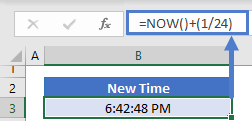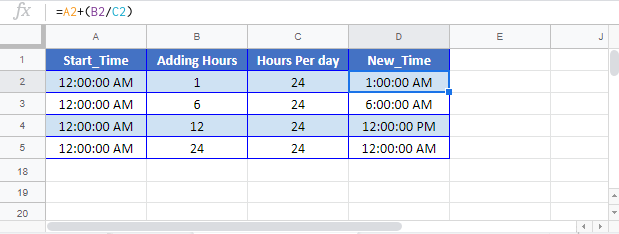This tutorial will demonstrate how to add hours to a time in Excel and Google Sheets.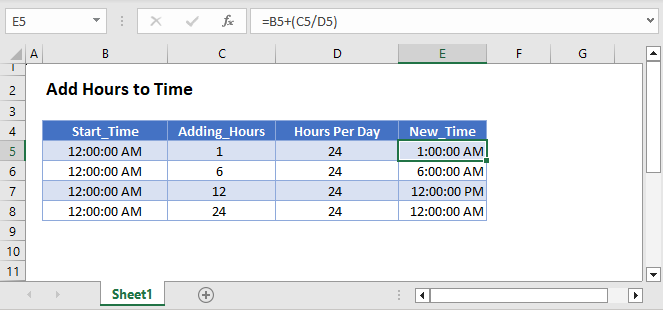In Excel, time is stored as a fractional value where each 1/24th represents one hour of the day.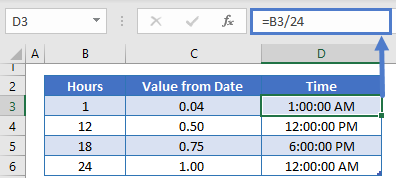``=B3+(1/24)``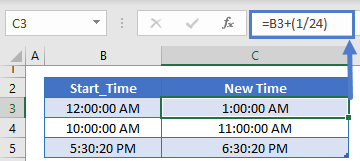or 12/24 to add 12 hours:

``=B3+(12/24)``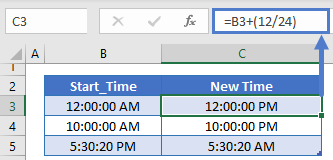To add an hour to the current time, add 1/24 to the NOW Function:

``=NOW()+(1/24)``